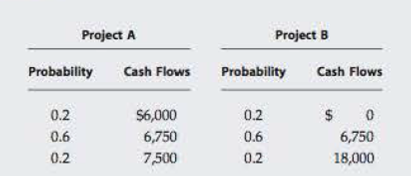Chapter 12, Problem 12PFundamentals of Financial Manageme...

14th Edition
Eugene F. Brigham + 1 other
ISBN: 9781285867977

Solutions

Chapter
SectionFundamentals of Financial Manageme...

14th Edition
Eugene F. Brigham + 1 other
ISBN: 9781285867977
Textbook Problem

PROJECT RISK ANALYSIS The Butler-Perkins Company (BPC) must decide between two mutually exclusive projects. Each costs $6,750 and has an expected life of 3 years. Annual project cash flows begin 1 year after the initial investment and are subject to the following probability distributions:BPC has decided to evaluate the riskier project at 12% and the less-risky project at 10%. a. What is each project's exported annual cash flow? Project B's standard deviation (σB) is$5,798, and its coefficient of variation (CVB) is 0.76. What are the values of σA and CVA? b. Based on the risk-adjusted NPVs, which project should BPC choose? c. If you knew that Project B's cash flows were negatively correlated with the firm's other cash flows, but Project A's cash flows were positively correlated, how might this affect the decision? If Project B's cash flows were negatively correlated with gross domestic product (GDP), while A's cash flows were positively correlated, would that influence your risk assessment?

a.

Summary Introduction

To compute: The projects expected annual cash flows, and compute the standard deviation and coefficient of variation of project A.

Introduction:

Project Risk Analysis:

The company should select a project that gives the maximum return and less risk. The process of analyzing the risk factor involved in the project is known as the project risk analysis. The management knows that almost every project involve risk element, so they select the lowest risky project among the options.

Explanation

Given information:

There are two mutually exclusive projects, A and B.

Cost of the project A and B is $6,750. B company evaluate risky project at 12% and less risky at 10%. Table shows expected annual cash flows of project A  Probability (X) Cash flows ($) (Y) Expected cash flows ($) (X)×(Y) 0.2 6,000 1,200 0.6 6,750 4,050 0.2 7,500 1,500 6,750 Table (1) Table shows expected annual cash flows of project B  Probability (X) Cash flows ($) (Y) Expected cash flows ($) (X)×(Y) 0.2 0 0 0.6 6,750 4,050 0.2 18,000 3,600 7,650 Table (2) Given, Standard deviation of project B is$5,798

Coefficient of variation of project B is 0.76

Calculation of standard deviation of project A,

 Year Expected cash flows(\$) (Z) (Q) (Y)−(Sum of (Z)

b.

Summary Introduction

To explain: The project to choose by the B Company on the basis of NPV.

c.

Summary Introduction

To explain: Effect on decision if Project B’s cash flows are negatively correlated with GDP.

Still sussing out bartleby?

Check out a sample textbook solution.

See a sample solution

The Solution to Your Study Problems

Bartleby provides explanations to thousands of textbook problems written by our experts, many with advanced degrees!

Get Started

Find more solutions based on key concepts